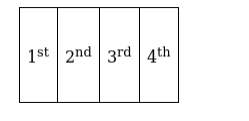# How many 4-digit numbers can be formed with the digits

Question:

How many 4-digit numbers can be formed with the digits 0, 2, 3, 4, 5 when a digit may be repeated any numbers of time in any arrangement?

Solution:

Let Suppose 4 digit number as 4 boxes as shown below. First Box is at

$1000^{\text {th }}$ place, the Second box is at $100^{\text {th }}$ place, the Third box is at $10^{\text {th }}$ place, and Fourth box is at $1^{\text {st }}$ place.The $1^{\text {st }}$ box can be filled with four numbers $(2,3,4,5)$ if we include 0 in the 1 stbox then it becomes 3 digit number(i.e. 0234 is 3 digit number, not 4 digits)

The $2^{\text {nd }}$ box can be filled with five numbers $(0,2,3,4,5)$ as repetition is allowed.

Similarly, the $3^{\text {rd }}$ box can be filled with five numbers $(0,2,3,4,5)$ as repetition is allowed.

Similarly, the $4^{\text {th }}$ box can be filled with five numbers $(0,2,3,4,5)$ as repetition is allowed.

Total number of ways is $4 \times 5 \times 5 \times 5=500$